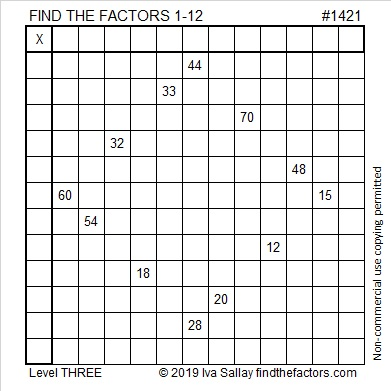# 1421 Square-shaped Sweet

This square-shaped puzzle is a Level 3 because you can start with clue 44 and work down the puzzle cell by cell to find the solution. You won’t get into a rhythm with this one, but logic will still help you to find the factors of the clues in order from top to bottom. That’s pretty sweet!Print the puzzles or type the solution in this excel file: 12 Factors 1419-1429

Now here are some sweet facts about the puzzle number 1421:

• 1421 is a composite number.
• Prime factorization: 1421 = 7 × 7 × 29, which can be written 1421 = 7² × 29.
• 1421 has at least one exponent greater than 1 in its prime factorization so √1421 can be simplified. Taking the factor pair from the factor pair table below with the largest square number factor, we get √1421 = (√49)(√29) = 7√29.
• The exponents in the prime factorization are 2 and 1. Adding one to each exponent and multiplying we get (2 + 1)(1 + 1) = 3 × 2 = 6. Therefore 1421 has exactly 6 factors.
• The factors of 1421 are outlined with their factor pair partners in the graphic below.1421 is also the hypotenuse of a Pythagorean triple:
980-1029-1421 which is (20-21-29) times 49.

This site uses Akismet to reduce spam. Learn how your comment data is processed.